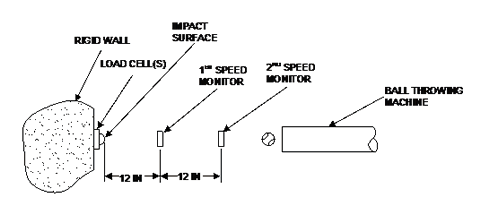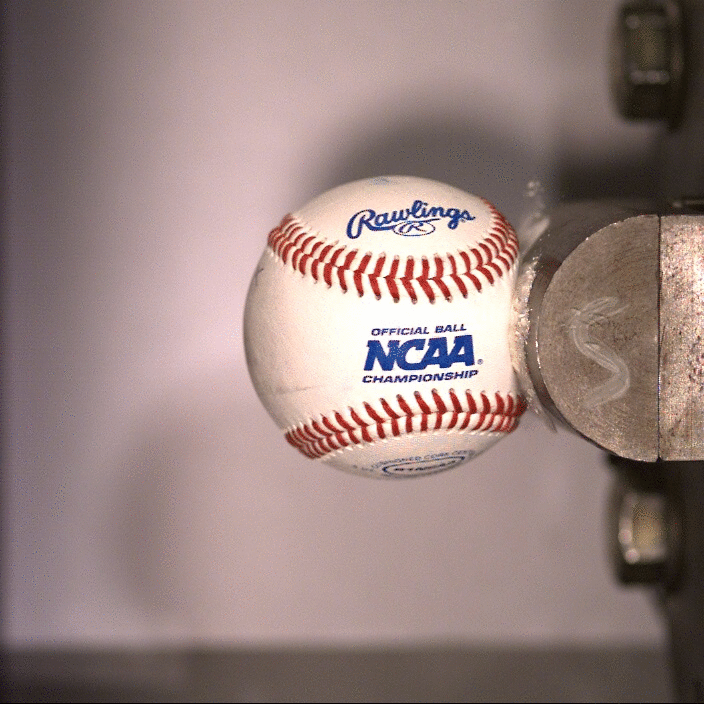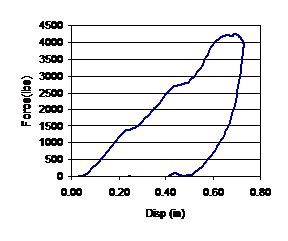Sports Science Laboratory Bat-Ball Science Dynamic Stiffness

## Dynamic Stiffness

Dynamic stiffness is a measure of ball stiffness, similar to compression. It is done against a cylindrical surface at a high rate of speed to simulate a bat impact. Test results show it correlates better with bat performance than compression.

In the compression test discussed above, one may observe that the 0.25 inches of displacement is applied diametrically, and produces only 0.125 inches of radial displacement. The ball can displace radially nearly one inch in a bat-ball collision, as shown in Fig. 4.1. The rate of deformation in the compression test is also much slower than occurs in a bat-ball collision (four orders of magnitude, or 10,000 times slower). This difference in the rate and magnitude of ball deformation limit the ability of ball compression to describe ball response in a bat-ball impact.

A test, depicted in Fig. 4.2, has been developed to better approximate the rate and magnitude of ball deformation occurring in a bat impact[c]. The test configuration is similar to that used to measure ball COR (Fig. 2.1). The displacement of the ball is comparable to the bat as shown in Fig. 4.3. An essential difference is that the impact surface is cylindrical (intended to approximate the bat) and load cells are used to measure the impact force. Ball COR can be obtained from this test, but it is lower than the standard ball COR. The lower COR value is due to the higher impact speed and the cylindrical impact surface. These tend to increase ball deformation which reduce ball COR. It is designated as CCOR to distinguish it from the standard measure of ball COR.Fig. 4.1. Photograph of a bat-ball impact at maximum displacementFig. 4.2. Schematic of dynamic stiffness test apparatus.

####Fig. 4.3. GIF of a ball impacting a rigid cylinder in a dynamic stiffness test.

Upon impact of the ball with the rigid cylinder, the load cells record the impact force as a function of time, Fig. 4.4. Knowing the incident ball speed, vi, and weight, m, the stiffness of the ball, k, may found from the peak impact force, F as(4.1)

The expression is obtained by equating the known kinetic energy of the incoming ball (½mv2) with its stored potential energy at maximum displacement (½kx2). The unknown ball displacement, x, is obtained from the peak impact force assuming the ball behaves as a linear spring (F=kx). This leaves only one unknown, k, representing the ball stiffness, Eq. (4.1).Fig. 4.4. Representative impact force reading during dynamic stiffness test.

A potential shortcoming of this approach is the assumption that the ball deforms as a linear spring. The accuracy of this assumption may be tested by examining a plot of the ball force-displacement during impact. Unfortunately the nature of the ball impact is unsuitable for a direct measure of ball displacement. An indirect measure of ball displacement can be obtained by integrating its acceleration twice. The acceleration, in turn, is found by dividing the impact force by the ball mass. A representative example of the ball force-displacement curve is presented in Fig. 4.5, which shows a relatively linear response during the loading phase. (Hysteresis and non-linearity during the unloading phase determine the energy loss and CCOR, but do not affect the linear spring assumption associated with the loading phase.)

The dynamic stiffness from 12 dozen softballs ranged between 6000 and 8000 lb/in while the CCOR ranged from 0.35 to 0.38.Fig. 4.5. Representative ball force-displacement curve during a dynamic stiffness test.

The dynamic stiffness test was developed to describe both the deformation rate and magnitude of a bat-ball impact. A relatively wide range of impact speeds would approximate the deformation rate reasonably well. To capture the deformation magnitude, however, the impact speed must be chosen more carefully. One might ask: at what speed should a rigid cylinder be impacted to simulate an impact with a recoiling bat? One plausible answer would be the speed where the impulse is the same for the two cases. Impulse is the area under the curve in Fig. 4.4, and is the momentum change at impact producing the rebound ball speed. Another plausible answer would be the speed where the ball deformation is the same for the two cases. A relation between the recoiling, vr, and fixed, vf, cases can be found as

where c=mQ2/I, and the exponent, n, is unity for constant impulse and ½ for constant deformation. (The other terms will be discussed in more detail later. For completeness, Q is the distance from the bat pivot to the impact location and I is the mass moment of inertia of the bat about the pivot point or MOI.) The constant deformation case correlates better with experimental measurements.

The test speed will depend on the impact location, Q, and bat MOI. For a bat-ball relative speed of 110 mph, this produces a rigid-wall test speed of 90 mph (light bat with an outside impact) to 100 mph (heavy bat with an inside impact). The test speed was selected as 95 mph to average between these extreme cases. In the test, the cylinder is impacted 6 times, from which the average dynamic stiffness is reported.

[c] Duris, J., Smith, L. V., 2004, “Evaluating Test Methods Used to Characterize Softballs,”  The Engineering of Sport 5th International Conference, Vol. 2, pp. 80-86, Davis, CA.